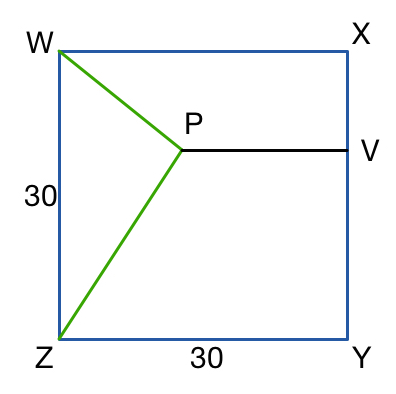# Proper Alignment

Geometry Level 3$WXYZ$ is a square of side length 30. $V$ is a point on $XY$ and $P$ is a point inside square with $PV$ perpendicular to $XY$, also $PW = PZ = PV-5$. What is $PV$?

×